# Blog

 This Site This List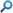## BlogOther Blogs
 There are no items in this list.MathsShare > Blog > Categories
 And while you are here...
... take a look at the home page MathsShare.com and like it!
 Crazy Curves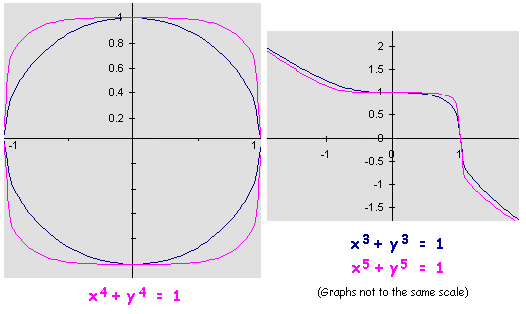Except for the circle (which is shown for reference), on the other three curves, except where the curves cross an axis, all the points (x,y) have an interesting property. In fact this property holds for all the curves of this family for any power of x and y above 2. What is so special about the co-ordinates (x,y) of these points and why?

 Curious numbers

I saw a tweet on my @SofARMaths account yesterday from Nalini Joshi @monsoon0 which read "I love all numbers great & small. Why are there so many anthropomorphic adjectives for them: odious, evil, perfect, nice, pernicious?" This prompted a frivolous exchange of tweets during which I asked whether there are any "curious" numbers.

I decided to call a curious number one that belongs to the set {Cn} where Cn is the product of the first n prime numbers +1. This is a definition that has been buzzing around my head for many months, probably years, since I first encountered Euclid's proof that the number of primes is infinite.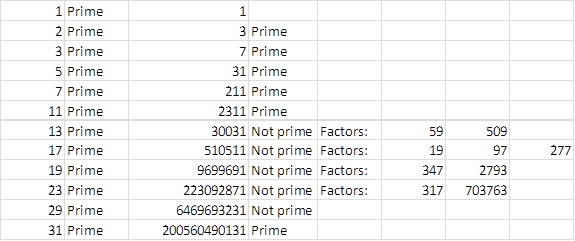When I asked myself whether 200560490131 is prime I came across a link to primorial numbers which shows that this area is already well researched. Oh well – perhaps we can classify these and other numbers by the number of prime factors they have. Is there a name for numbers which are the product of exactly 2 prime numbers such as 30031?

 Binary patterns in the primes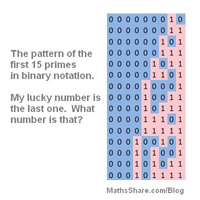Don’t know why but after following several tweets under the #bbccode hashtag I was thinking about the patterns in the primes and decided to ask myself what patterns there might be in a binary listing of the primes.  Prime numbers (and Fermat’s last theorem) always seem to pop into my head at random moments – this time on Wimbledon station.

I mentioned my thoughts “@SofARMaths wondering if you write primes in binary one beneath the other what pattern do you get.” And received a reply from @bbccode “@SofARMaths great idea! give it a try and send us a picture!”  Well here is the pattern for the first 97 primes. (click here)

Let me know what you make of it...

 Vector proof – Join the mid-points of the sides of any quadrilateral to form a parallelogram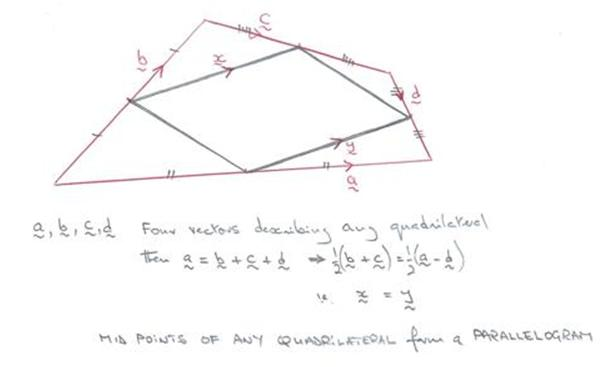Construct the square root of a number

I have been struggling to remember how first I came up with a construction for the puzzle: "Square root Draw a line x cms long. Now using ruler and compass only construct a line whose length is the square root of x." The original idea came from considering the ladder puzzle. However the construction below relies only on the application of Pythagoras' Theorem as you may be able to see from my brief hand written explanation below (where, I have just noticed, I have used y for the length of the line instead of x as quoted in the puzzle!)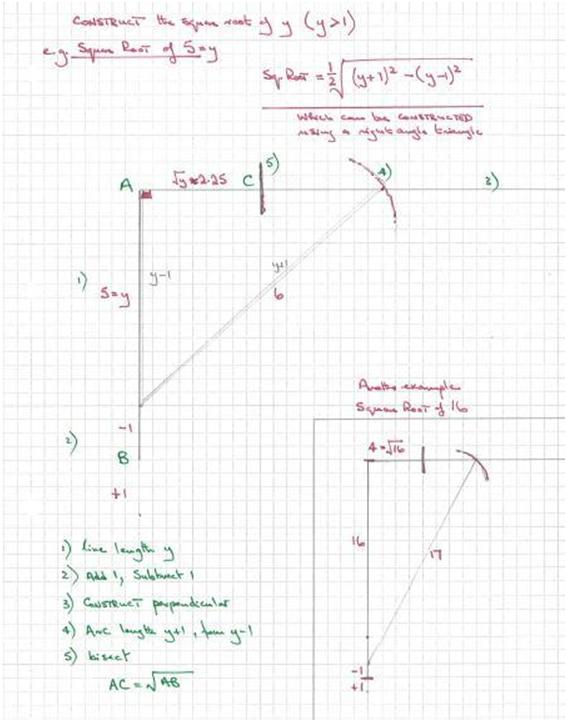Mirror a point in a line - Oxford Mathematics entrance question

The normal to the line ax + by = c is the vector (a,b).
Hence the normal to the line 3x + 4y = 50 is (3,4).

Any point from (x1,y1) on a normal is of the form (x1,y1) + k(a,b) – which is, if I remember rightly, (3,4) + k(3,4) in the example question.  I think only (9,12) on the list of possible answers will fit? (with k=2).